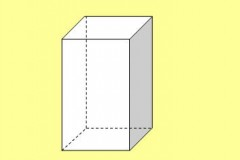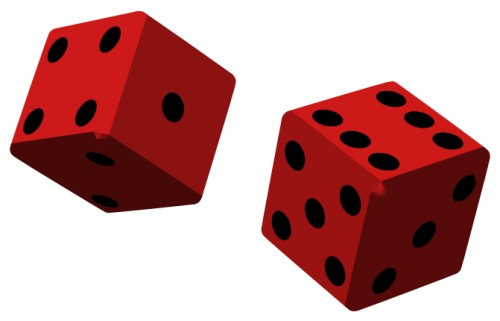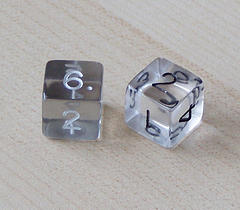# How Many Faces, Edges, Vertices Does A Rectangular Prism Have?

## 30 Answers

Anonymous answered
A rectangular Prism has 6 faces, 12 edges, and 8 vertices

A face is a flat surface of a solid - it doesn't matter if it is side, top or bottom
an Edge is anywhere where two faces meet - basically count your lines
the Vertices is basically your corner - where 3 edges meet

So the rectangular Prism is 6, 12, 8
thanked the writer.
Anonymous commented
Shapes are cool!!!!!!!!!!!!!!
Mark Mottian answered
A rectangular prism or a cuboid has:

• 6 faces
• 12 edges
• 8 verticesthanked the writer.
Anonymous answered
6 faces 12 edges 8 vertices.
thanked the writer.
Anonymous answered
6 faces,12 edges,8 vertices
thanked the writer.
Anonymous answered
It has 6 faces 12 vertices and 8 edges
thanked the writer.
Anonymous answered
8 edges  6 faces
thanked the writer.
Anonymous answered
4 base 6 Faces 12 Edges 7 Vertices
thanked the writer.
Anonymous answered
6 faces, 8 vertices, and 12 edges
thanked the writer.
Anonymous answered
5 faces
9 edges
5 vertices
thanked the writer.
9 edges,5 faces,6 vertices/corners
thanked the writer.
Anonymous answered
4 edges, 5 vertices, and 5 faces.   :-D
thanked the writer.
Anonymous answered
5 faces 8 edges and 5 vertices
thanked the writer.
Anonymous answered
9 edges      6  vertices    5  faces
thanked the writer.
Anonymous answered
6 faces,8 edges, 8 vertices's
thanked the writer.
Anonymous answered
8 vertices are on the pyramid
thanked the writer.
5 faces

8 edges

5 vertices
thanked the writer.
Anonymous answered
The faces is 12,edges is 12.
thanked the writer.
Anonymous answered
4 faces 3 edges 2 vertices
thanked the writer.
Anonymous answered
6 faces, 2 edges, and 5 corners
thanked the writer.
6,19, and10,000,000

thanked the writer.
Ty ########## answered
A rectangular prism has 4 faces and a bottom, 8 edges and 0 verticals
thanked the writer.
Anonymous answered
4 faces 8 edges 0 vertices
thanked the writer.
Lee answered
Actually it's:

9 edges
6 vertices/corners
5 faces
thanked the writer.
Anonymous answered
4 faces  4 edges  1 vertices
thanked the writer.
Oddman answered
In this picture, they are numbered for you.thanked the writer.
Anonymous answered
It Has,
9 vertices
6 faces
4 edges
thanked the writer.
lance holder answered
It has 5 faces, it has 9 edges, and it has 6 vertices or corners
thanked the writer.
Anonymous answered
5. Picture a rectangle with a point at the top. 1, 2, 3, 4, vertices has a rectangle and then the point, 5.
thanked the writer.
Oddman answered
A rectangular prism is the solid 3-dimensional geometric figure resulting from translating a rectangle in a direction perpendicular to the plane in which it lies.It has six (6) flat surfaces, called faces. These are the original rectangle (the base), the translated rectangle (another base), and the surfaces that result from translating each of the rectangle's four (4) sides (the lateral faces). On a die, or "number cube", the faces are numbered.

Each base has four (4) corners. These comprise the eight (8) vertices of the figure. The four edges of each base, and the four edges joining the two bases comprise the 12 edges of a rectangular prism.

Rectangular prisms come in many forms and sizes, including the tiniest computer chips, gaming dice, construction bricks and blocks, shipping boxes, and concrete runways miles long and feet thick.
thanked the writer.
Anonymous answered
Faces 4
vertices 0
Edges 8
Base 2
thanked the writer.

## Answer Question